# Smith Chart 史密斯圖 怎麼用-高頻電路的好幫手

## 傳輸線 Transmission Line

$$\Gamma (l) =\frac{V_{reflected}}{V_{incient}}=\frac{Z_{L}-Z_{0}}{Z_{L}+Z_{0}}e^{-j2\beta l} =\Gamma _{0}e^{-j2\beta l}$$

$$Z_{L}$$是負載阻抗、$$Z_{0}$$是特徵阻抗、$$l$$是傳輸線長度，至於 $$\beta$$則是角波數，它的概念與時域的角頻率$$w$$是一樣的，但頻率這個詞已經被時域用掉了，所以電壓在空間距離上的變化我們稱為波數，詳情可以參考這篇波長與週期-到底誰是誰-別搞混了。$$\beta$$與$$Z_{0}$$是材料特性，不同材料會有不同的值。

## 特徵阻抗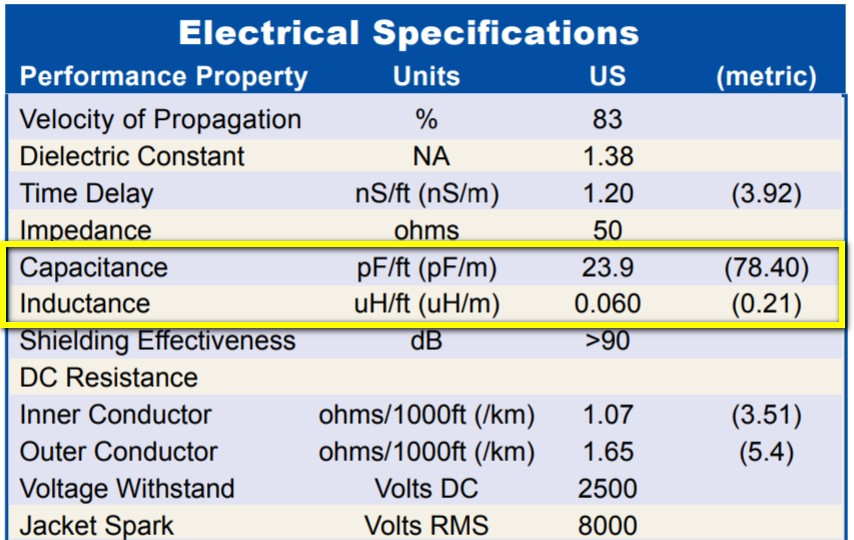LMR-400 cable spec

$$Z_{0}=\sqrt{\frac{L}{C}}$$

## 導線長度與反射率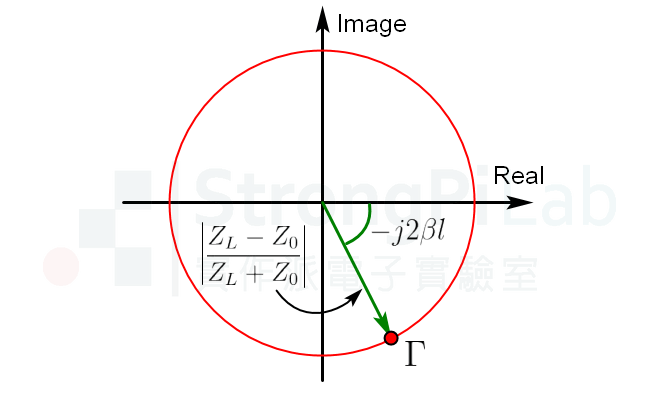反射率在複數平面的極座標形式

• $$\Gamma_{0}=-1$$ 表示負載短路Short
• $$\Gamma_{0}=0$$ 表示負載阻抗匹配Impedance Match，無反射
• $$\Gamma_{0}=1$$ 表示負載開路

## Smith Chart 史密斯圖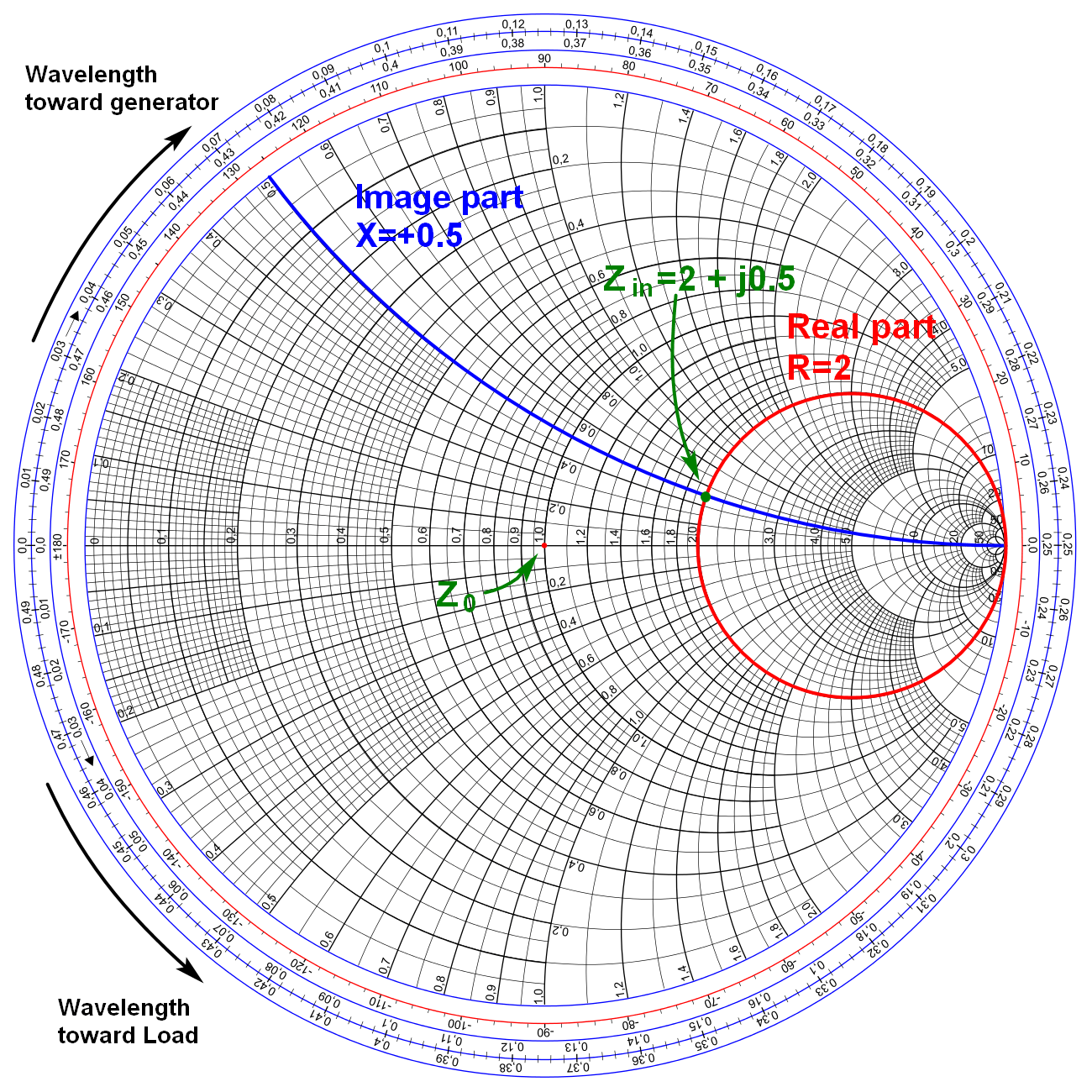史密斯圖 Smith Chart 正規化版本

## 快速計算導納

$$Y=\frac{1}{Z}=G+jB\; (S)$$阻抗與導納合併的Smith Chart 50 ohm版本

## 傳輸線長度傳輸線長度增加會讓反射率順時針旋轉

## 阻抗匹配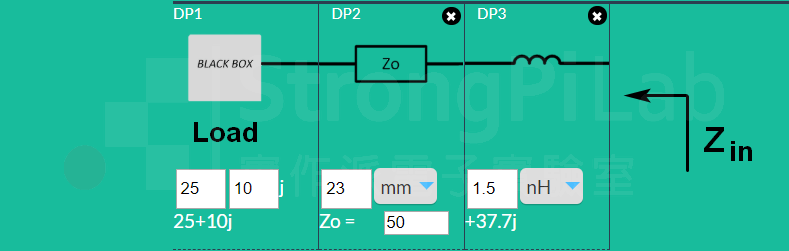阻抗匹配線路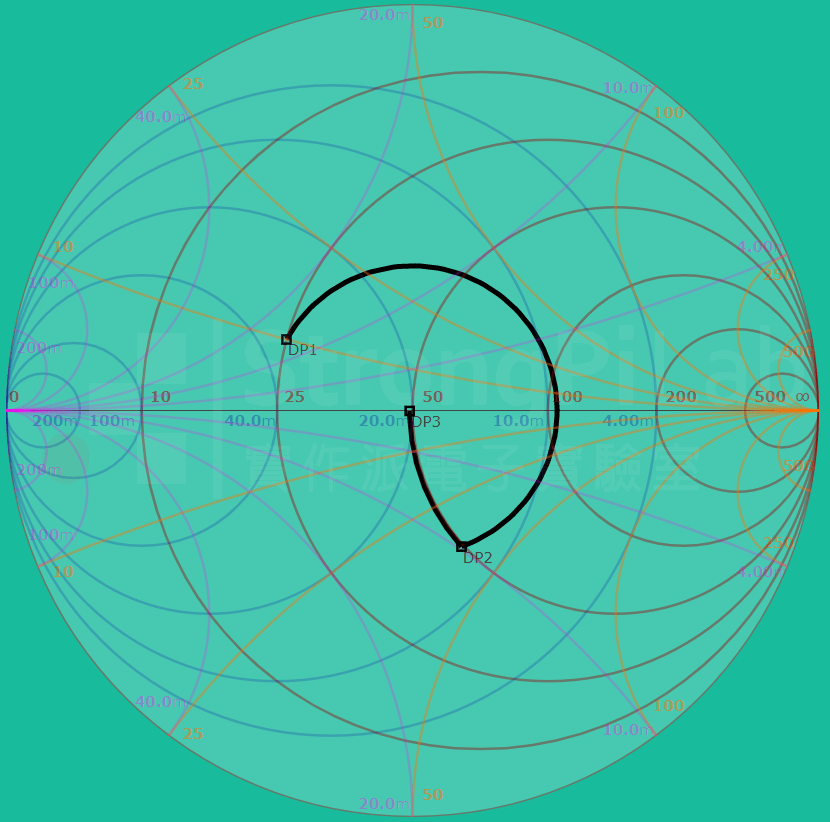在Smith Chart做阻抗匹配在Smith Chart上的頻率響應

## 相關文章

#### 7 Comments

1.alexseo表示:

了解了
謝謝大大回答
網站中的文章內容受益良多!!
謝謝!

2.alex seo表示:

大大你好
請問在阻抗匹配的章節中
DP3的電感
除了1.5nH
他下面還有一個+37.7j
請問這個數字是什麼意思呢?

•阿信助教表示:

串聯了1.5nH的電感之後, 會增加的阻抗
Z=jwL, 你把值套進去算一算就會得到37.7j ohm

3.Abel Fong表示:

Good job!!! The contents are well explained all the details and how Smith chart works. Thanks for sharing.

•阿信助教表示:

Thanks for your feedback

4.jeffrey表示:

越靠近2G Hz就越靠近中心的匹配點, 是否應該為4G hz?另外Y=20m−j20m
,為何從smith chart上看,虛部是-20

•阿信助教表示:

感謝您的提醒, 確實是4GHz才對, 我會更正
Y的部分, smith chart上面Y虛部的數值後面有跟著m
可能不是很顯眼, 所以容易忽略